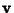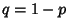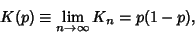## s-Run

N.B. A detailed on-line essay by S. Finch was the starting point for this entry.

Letbe a-Vector whose entries are each 1 (with probability) or 0 (with probability). An-run is an isolated group ofconsecutive 1s. Ignoring the boundaries, the total number of runssatisfiessowhich is called the Mean Run Count Per Site or Mean Run Density in Percolation Theory.

Finch, S. Favorite Mathematical Constants.'' http://www.mathsoft.com/asolve/constant/rndprc/rndprc.html